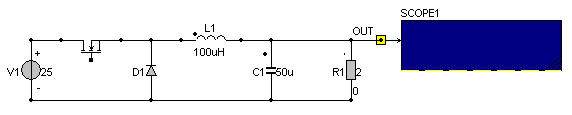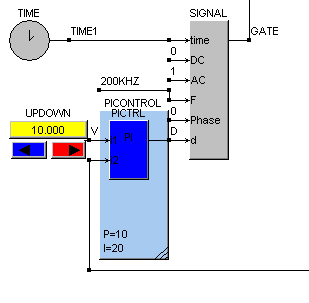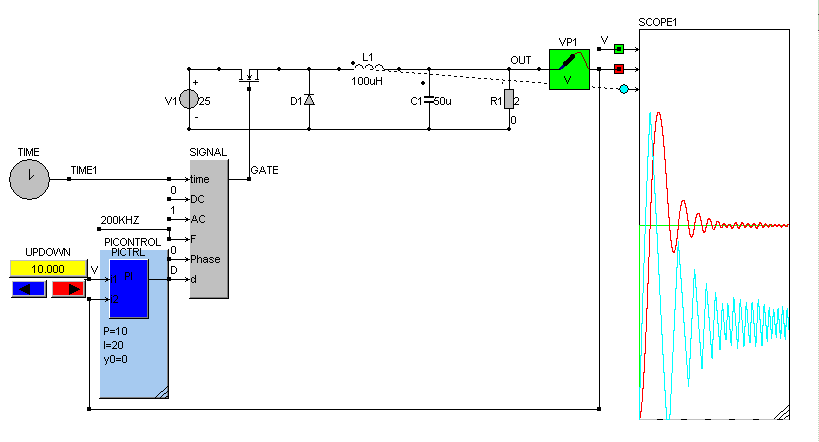• Introduction
• What is in this manual
• What is Caspoc
• User interface
• Introduction
• Starting
• Simulation
• Editing
• Viewing and printing
• Getting Started
• Basic editing
• Simulation in the time domain
• Basic User Interface Topics
• Editing
• Simulation
• Viewing
• Library
• Reports
• Project management
• Circuit and Block Diagram Components
• Introduction
• Cscript and user defined functions
• Component parameters
• Modeling Topics
• Introduction
• Power Electronics
• Semiconductors
• Electrical Machines
• Electrical drives
• Power Systems
• Mechanical Systems
• Thermal Systems
• Magnetic Circuits
• Green Energy
• Coupling to FEM
• Experimenter
• Analog hardware description language
• Embedded C code Export
• Coupling to Spice
• Small Signal Analysis
• Matlab coupling
• Tips and tricks
• Appendices

## PI Control for a buck converter.

The buck converter from the previous tutorial is extended with a PI control. We will create a model where the mosfet is controlled from the block diagram where the PI controlled is modeled. Create a circuit as shown below:The gate of the Mosfet is a block diagram node. This is indicated by the square node. We will control the gate of the Mosfet by using a SIGNAL block. The block SIGNAL is used to create wave forms like square waves, sinusoidal and triangular waveforms. Select the block TIME and SIGNAL from Components/Blocks/SourceThe block SIGNAL is used for creating the square wave form for controlling the gate. Open the block SIGNAL by clicking it with the right mouse button. The third parameter indicates the type of the signal. There are various wave forms possible such as sinusoidal, square wave, triangular, etc. Default the third parameter is set to 1 to select a square wave form.

Please note that in most cases the first input has to be connected to the block Time. The DC value of the source is set to 0 and the AC value is set to 1. The frequency of the square wave form is set to 200kHz with a phase-shift of 0 rad. In the previous tutorial the duty cycle, being the last input of the block SIGNAL was set to 0.5. In this control a PI control is used with the following parameters:

• P=10
• I=20
The first input to the PI control is the reference voltage. The reference voltage comes from an interactive UPDOWN block. During the simulation the user can change the value of the output of this block by clicking the blue or red buttons. The secon input is the output voltage of the buck converter. Use a voltage probe VP that is selected from Components/Library/Probes to measure the output voltage.

The gate of the Mosfet is connected to the output of the block SIGNAL. The simulation is shown below.